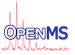OpenMS  2.8.0
ContinuousWaveletTransformNumIntegration Class Reference

This class computes the continuous wavelet transformation using a marr wavelet. More...

`#include <OpenMS/TRANSFORMATIONS/RAW2PEAK/ContinuousWaveletTransformNumIntegration.h>`

Inheritance diagram for ContinuousWaveletTransformNumIntegration:
[legend]
Collaboration diagram for ContinuousWaveletTransformNumIntegration:
[legend]

## Public Types

typedef ContinuousWaveletTransform::PeakConstIterator PeakConstIterator
Profile data const iterator type. More...Public Types inherited from ContinuousWaveletTransform
typedef std::vector< Peak1D >::const_iterator PeakConstIterator
Raw data const iterator type. More...

## Public Member Functions

ContinuousWaveletTransformNumIntegration ()
Constructor. More...

~ContinuousWaveletTransformNumIntegration () override
Destructor. More...

template<typename InputPeakIterator >
void transform (InputPeakIterator begin_input, InputPeakIterator end_input, float resolution)
Computes the wavelet transform of a given profile data interval [begin_input,end_input) More...

void init (double scale, double spacing) override
Perform necessary preprocessing steps like tabulating the Wavelet. More...Public Member Functions inherited from ContinuousWaveletTransform
ContinuousWaveletTransform ()
Constructor. More...

virtual ~ContinuousWaveletTransform ()
Destructor. More...

const std::vector< Peak1D > & getSignal () const

std::vector< Peak1D > & getSignal ()

void setSignal (const std::vector< Peak1D > &signal)

const std::vector< double > & getWavelet () const

std::vector< double > & getWavelet ()

void setWavelet (const std::vector< double > &wavelet)

double getScale () const

double & getScale ()

void setScale (double scale)

double getSpacing () const

double & getSpacing ()

void setSpacing (double spacing)

SignedSize getSignalLength () const

SignedSizegetSignalLength ()

void setSignalLength (const SignedSize signal_length)

int getSize () const

double operator[] (unsigned int i)
Yields the signal (intensity) at position i. More...

double operator[] (unsigned int i) const

## Public Attributes

std::vector< Peak1Dsignal_
The transformed signal. More...

std::vector< double > wavelet_
The pre-tabulated wavelet used for the transform. More...

double scale_
Spacing and scale of the wavelet and length of the signal. More...

double spacing_

SignedSize signal_length_

## Protected Member Functions

template<typename InputPeakIterator >
double integrate_ (InputPeakIterator x, InputPeakIterator first, InputPeakIterator last)
Computes the convolution of the wavelet and the profile data at position x with resolution = 1. More...

double integrate_ (const std::vector< double > &processed_input, double spacing_data, int index)
Computes the convolution of the wavelet and the profile data at position x with resolution > 1. More...

double marr_ (const double x) const
Computes the Marr wavelet at position x. More...Protected Member Functions inherited from ContinuousWaveletTransform
template<typename InputPeakIterator >
double getInterpolatedValue_ (double x, InputPeakIterator it_left)Protected Attributes inherited from ContinuousWaveletTransform
std::vector< Peak1Dsignal_
The transformed signal. More...

std::vector< double > wavelet_
The pre-tabulated wavelet used for the transform. More...

double scale_
Spacing and scale of the wavelet and length of the signal. More...

double spacing_

SignedSize signal_length_

## Detailed Description

This class computes the continuous wavelet transformation using a marr wavelet.

The convolution of the signal and the wavelet is computed by numerical integration.

## ◆ PeakConstIterator

Profile data const iterator type.

## ◆ ContinuousWaveletTransformNumIntegration()

 ContinuousWaveletTransformNumIntegration ( )
inline

Constructor.

## ◆ ~ContinuousWaveletTransformNumIntegration()

 ~ContinuousWaveletTransformNumIntegration ( )
inlineoverride

Destructor.

## ◆ init()

 void init ( double scale, double spacing )
overridevirtual

Perform necessary preprocessing steps like tabulating the Wavelet.

Build a Marr-Wavelet for the current spacing and scale. We store the wavelet in the vector<double> wavelet_;

We only need a finite amount of points since the Marr function decays fast. We take 5*scale, since at that point the wavelet has dropped to ~ -10^-4

Reimplemented from ContinuousWaveletTransform.

## ◆ integrate_() [1/2]

 double integrate_ ( const std::vector< double > & processed_input, double spacing_data, int index )
protected

Computes the convolution of the wavelet and the profile data at position x with resolution > 1.

## ◆ integrate_() [2/2]

 double integrate_ ( InputPeakIterator x, InputPeakIterator first, InputPeakIterator last )
inlineprotected

Computes the convolution of the wavelet and the profile data at position x with resolution = 1.

References OpenMS::Math::round().

## ◆ marr_()

 double marr_ ( const double x ) const
inlineprotected

Computes the Marr wavelet at position x.

## ◆ transform()

 void transform ( InputPeakIterator begin_input, InputPeakIterator end_input, float resolution )
inline

Computes the wavelet transform of a given profile data interval [begin_input,end_input)

• Resolution = 1: the wavelet transform will be computed at every position of the Profile data,
• Resolution = 2: the wavelet transform will be computed at 2x(number of Profile data positions) positions (the profile data are interpolated to get the intensity for missing positions)
Note
The InputPeakIterator should point to a Peak1D or a class derived from Peak1D.
Before starting the transformation you have to call the init function

References OpenMS::Constants::k.

## Member Data Documentation

We often have to pad the transform at the left and right with zeros. Since we don't want to iterate over those as well, we have to store their positions.

## ◆ scale_

 double scale_

Spacing and scale of the wavelet and length of the signal.

## ◆ signal_

 std::vector signal_

The transformed signal.

## ◆ signal_length_

 SignedSize signal_length_

## ◆ spacing_

 double spacing_

## ◆ wavelet_

 std::vector wavelet_

The pre-tabulated wavelet used for the transform.